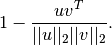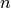# scipy.spatial.distance.cosine¶

scipy.spatial.distance.cosine(u, v)

Computes the Cosine distance between two n-vectors u and v, which is defined asParameters : u : ndarray An-dimensional vector. v : ndarray An-dimensional vector. d : double The Cosine distance between vectors u and v.

#### Previous topic

scipy.spatial.distance.correlation

#### Next topic

scipy.spatial.distance.dice